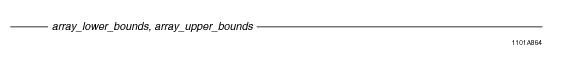# 15.00 - ARRAY Scope Reference - Teradata Database

## Teradata Database SQL Functions, Operators, Expressions, and Predicates

Product
Release Number
15.00
Content Type
Programming Reference
Publication ID
B035-1145-015K
Language
English (United States)

## ARRAY Scope Reference

### Purpose

References one or more elements of an ARRAY data type when invoking an ARRAY function.

### Syntaxwhere:

 Syntax element… Specifies… array_lower_bounds an integer value or an array instance of the predefined ARRAY type ArrayVec, with a comma-separated list of integer values to define the lower bounds. array_upper_bounds an integer value or an array instance of the predefined ARRAY type ArrayVec, with a comma-separated list of integer values to define the upper bounds.

### ANSI Compliance

This is a Teradata extension to the ANSI SQL:2011 standard.

### Usage Notes

To reference one or more elements of an ARRAY data type when invoking an ARRAY function, you can use an integer value for 1-D arrays, but you must use the ArrayVec ARRAY data type for n-D arrays. The ArrayVec ARRAY data type is automatically created by the system and is defined as:

`   CREATE TYPE ArrayVec AS INTEGER ARRAY; `

For all ARRAY functions that contain an optional scope_reference or array_bound argument, you can use one or more instances of the ArrayVec ARRAY type to describe the boundaries of each dimension of an n-D ARRAY data type.

### Rules

When the optional scope_reference parameter is specified in an ARRAY function, the following rules apply:

• You can pass two integer values or two ArrayVec values for the scope_reference. If the ARRAY function is called with one-dimensional ARRAY arguments, you can pass a combination of integer and ArrayVec values for the scope_reference. For example, the scope_reference can be <integer_value, ArrayVec_value> or <ArrayVec_value, integer_value>.
• If you use an ArrayVec instance for specifying the upper or lower bounds of a scope_reference, the following are not allowed:
• Passing in NULL for any of the ArrayVec elements that are needed to determine the bound of the array argument.
• Passing an insufficient number of elements in the ArrayVec to determine the bound of the array argument.
• Passing too many elements in the ArrayVec to determine the bound of the array argument.
• For all ARRAY functions that define the RETURNS NULL ON NULL INPUT clause, if one of the optional parameters to define the scope_reference is passed as NULL, then NULL is returned as the result value.
• ARRAY functions that are called with a scope_reference specified are considered to have their scope explicitly defined, so these functions return an error if any uninitialized elements fall within the range of the scope_reference in any of the ARRAY input arguments. The CARDINALITY function is an exception to this rule because an explicit scope that includes uninitialized elements is an acceptable input to the function.
• If your application requires operations between 2 arrays, consider creating these arrays using the DEFAULT NULL clause at creation time. This will prevent the system from returning errors due to illegal element references since all elements of the array will be initialized.

When the optional scope_reference parameter is not specified in an ARRAY function, the following rules apply:

• If you call an ARRAY function without specifying a scope_reference, the default scope used is the number of initialized elements in the input ARRAY argument. If an ARRAY function accepts two ARRAY arguments and the number of initialized elements is different between the two arrays, then an error is returned.
• When an ARRAY function returns an ARRAY value, the resultant ARRAY value will have the same number of initialized elements as the input ARRAY argument.
• ### Example

The following query returns the number of distinct elements within the range from 5 to 10 on each dimension of the phonelist array.

`SELECT CARDINALITY(phonelist, NEW ArrayVec(5,5), NEW ArrayVec(10,10));`